Polygons

Chapter 3 Class 8 Understanding Quadrilaterals
Concept wise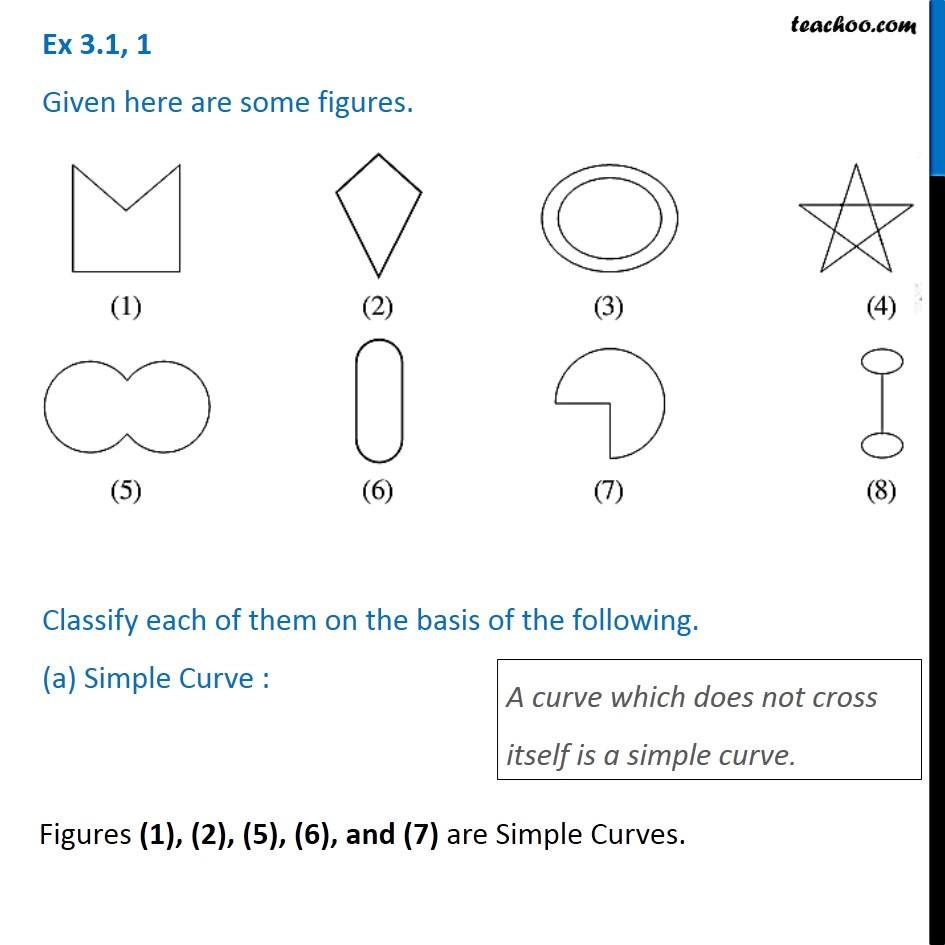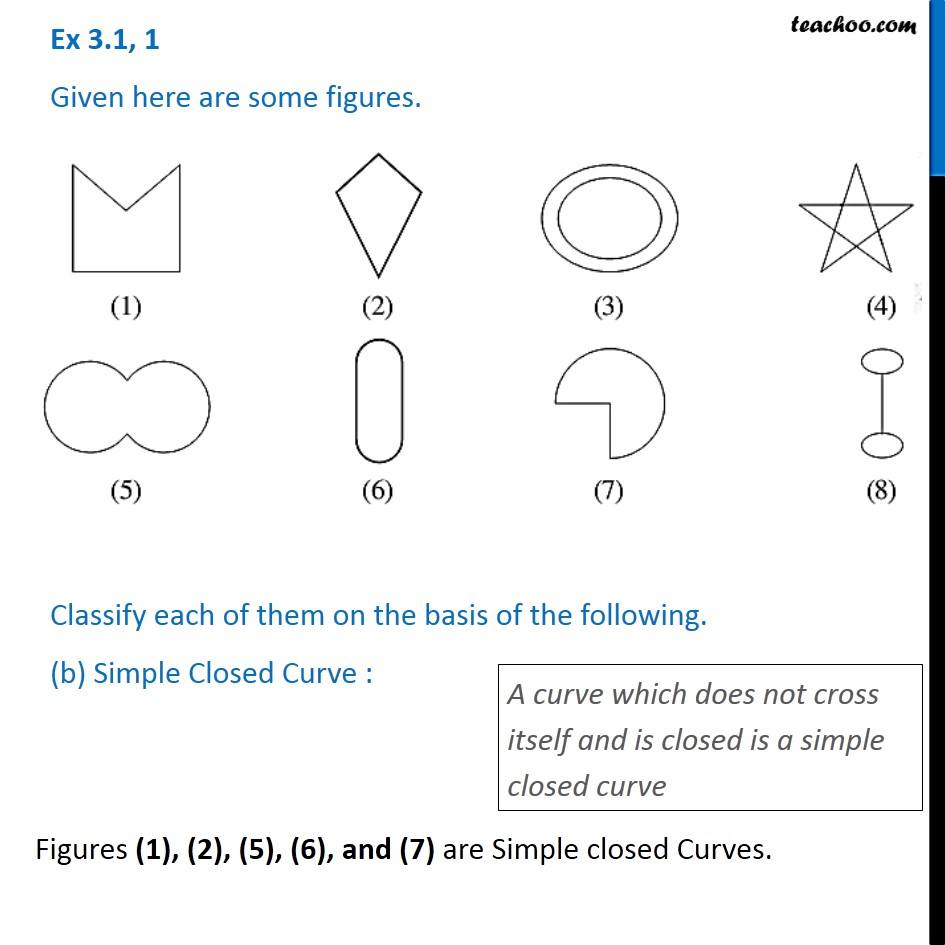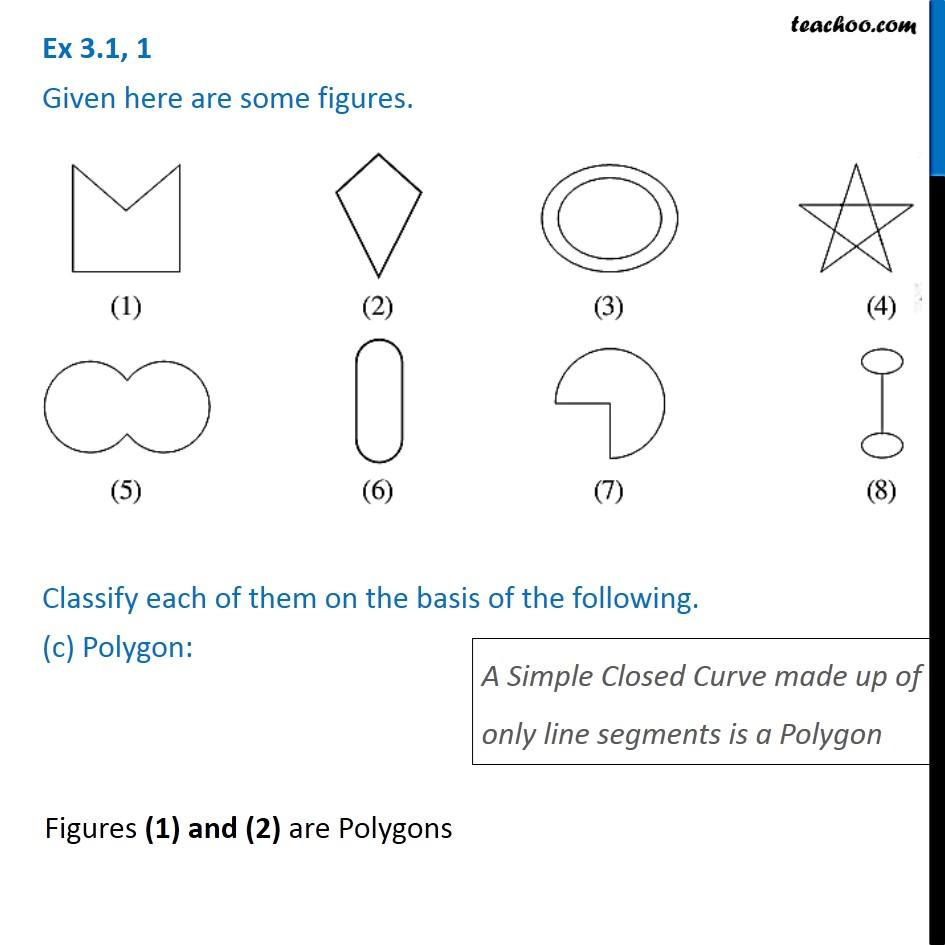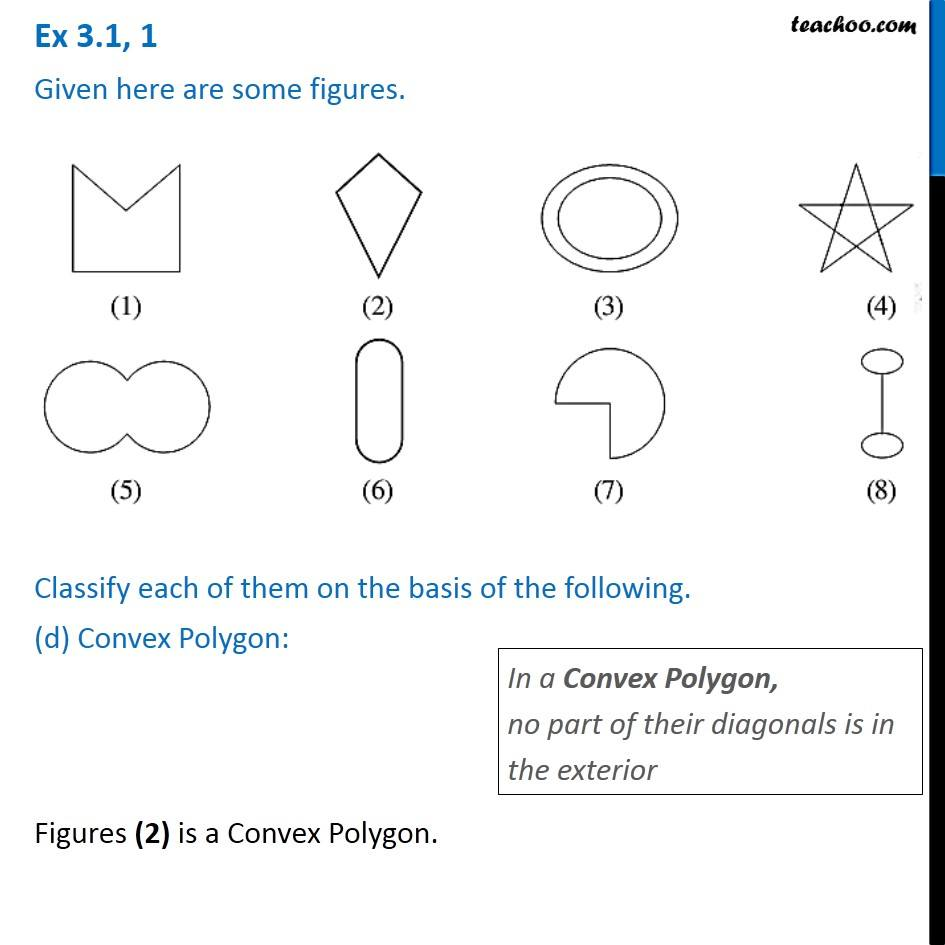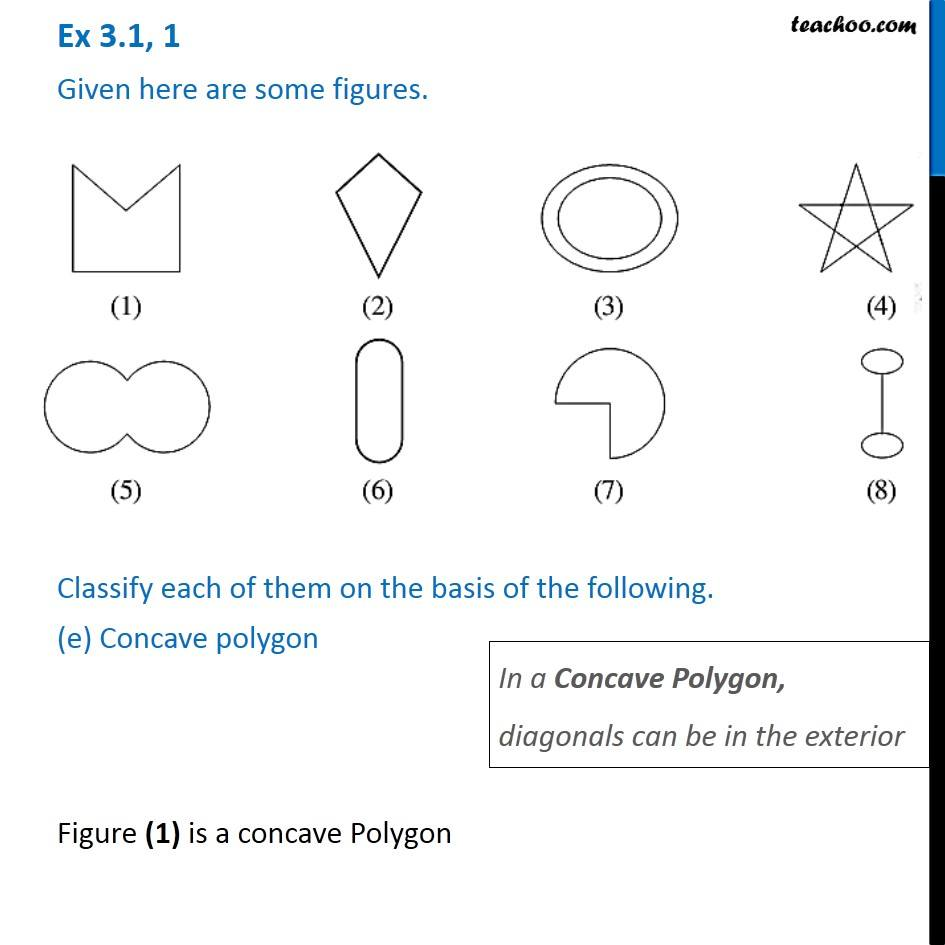Learn in your speed, with individual attention - Teachoo Maths 1-on-1 Class

### Transcript

Ex 3.1, 1 Given here are some figures. Classify each of them on the basis of the following. (a) Simple Curve : A curve which does not cross itself is a simple curve. Figures (1), (2), (5), (6), and (7) are Simple Curves. Ex 3.1, 1 Given here are some figures. Classify each of them on the basis of the following. (b) Simple Closed Curve : A curve which does not cross itself and is closed is a simple closed curve Figures (1), (2), (5), (6), and (7) are Simple closed Curves. Ex 3.1, 1 Given here are some figures. Classify each of them on the basis of the following. (c) Polygon: A Simple Closed Curve made up of only line segments is a Polygon Figures (1) and (2) are PolygonsFigures (1) and (2) are PolygonsIn a Convex Polygon, no part of their diagonals is in the exterior Figures (2) is a Convex Polygon. Ex 3.1, 1 Given here are some figures. Classify each of them on the basis of the following. (e) Concave polygon In a Concave Polygon, diagonals can be in the exterior Figure (1) is a concave Polygon